Question

Consider a projectile of mass 20 kg launched with a speed 9 m/s at an elevation angle of 45 degrees. Taking the launch point as the origin of your coordinate system---

What is the magnitude of the angular momentum at launch? kg m/s

What is the magnitude of the angular momentum at the instant it reaches maximum height? kg m/s

What is the magnitude of the angular momentum at impact?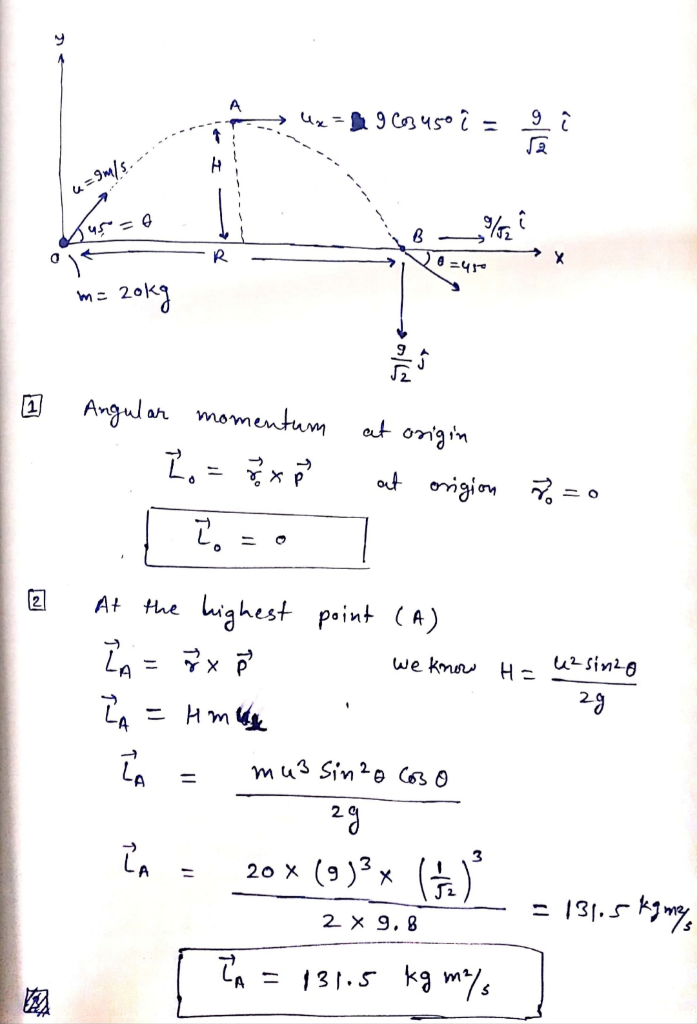9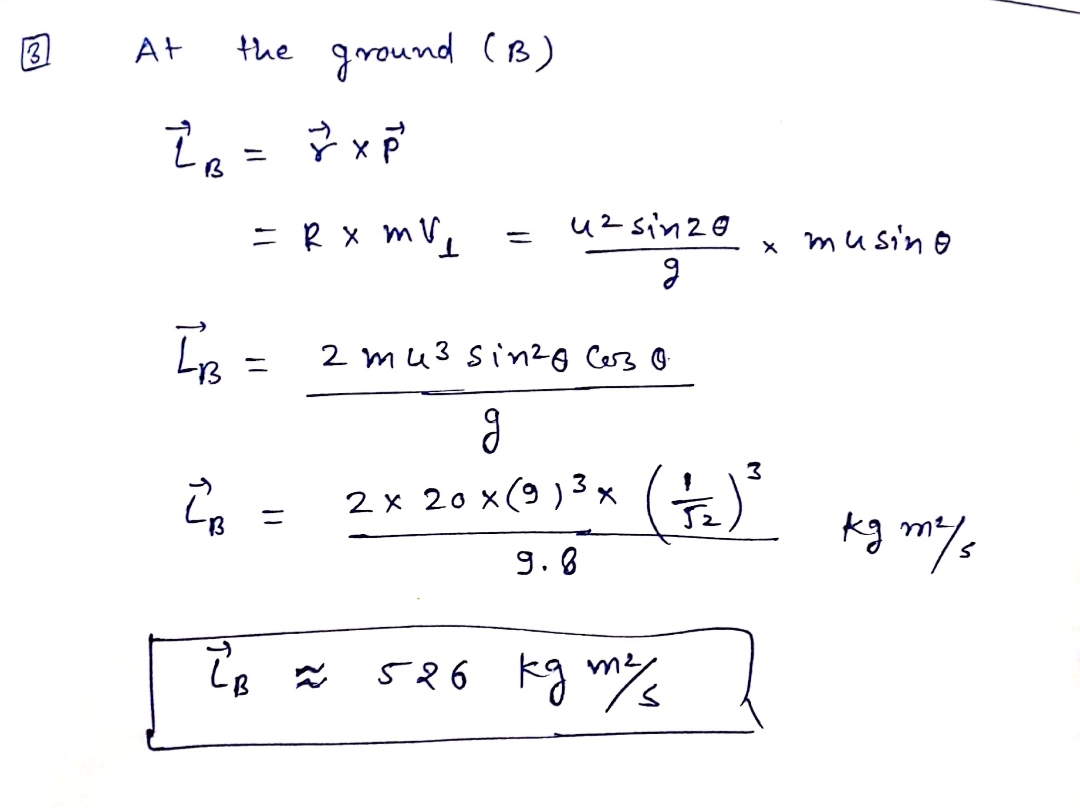#### Earn Coins

Coins can be redeemed for fabulous gifts.

Similar Homework Help Questions
• ### A projectile is launched from the top of a building at a height 15.4 m at...

A projectile is launched from the top of a building at a height 15.4 m at an angle of 37.4 degrees above horizontal and a launch speed of 25.5 m/s. The building is situated on flat level land. What will be the maximum height above the ground that the projectile reaches?

• ### A projectile with a mass of 8 kg is launched from the ground at 23 m/s...

A projectile with a mass of 8 kg is launched from the ground at 23 m/s at an angle of 30 degrees above the horizontal. As it travels through the air, the drag force (see problem 1) does -39 Joules of work on the projectile as it travels to its maximum height. What maximum height does the projectile reach, in meters?

• ### Problem 7 4.A projectile of mass 9.6 kg is launched from the ground with an initial velocity of 12.4 m/s at an angl...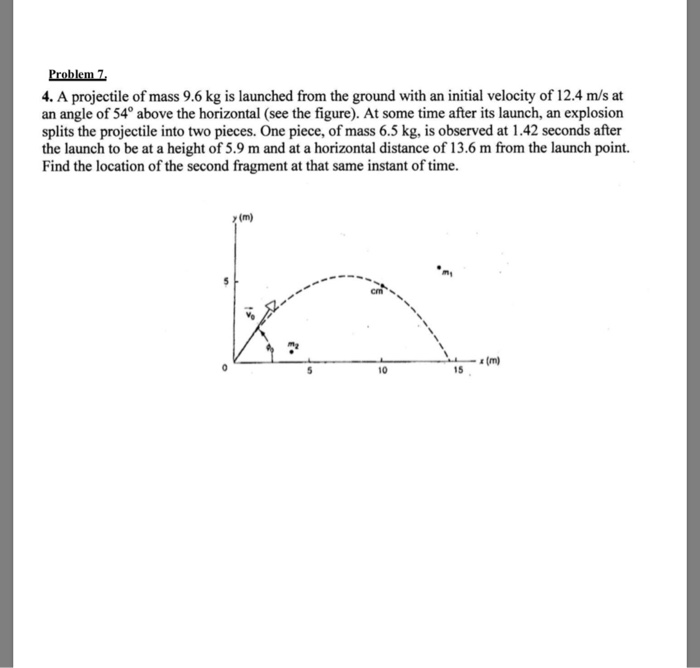Problem 7 4.A projectile of mass 9.6 kg is launched from the ground with an initial velocity of 12.4 m/s at an angle of 54° above the horizontal (see the figure). At some time after its launch, an explosion splits the projectile into two pieces. One piece, of mass 6.5 kg, is observed at 1.42 seconds after the launch to be at a height of 5.9 m and at a horizontal distance of 13.6 m from the launch point. Find...

• ### A projectile of mass M #13.4 kg is launched over level ground at a speed 28.6...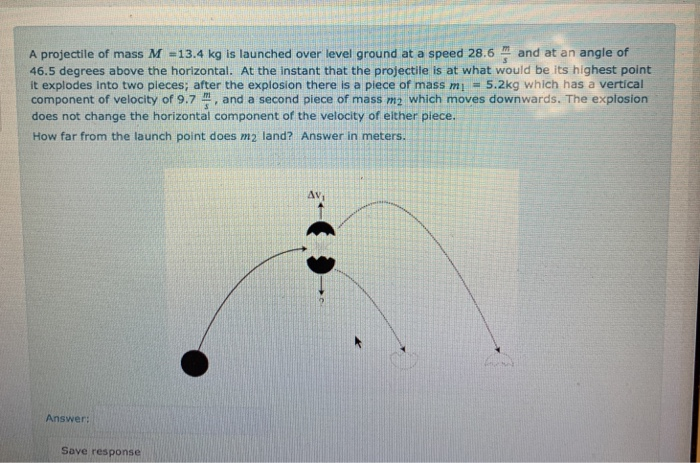A projectile of mass M #13.4 kg is launched over level ground at a speed 28.6 and at an angle of 46.5 degrees above the horizontal. At the instant that the projectile is at what would be its highest point it explodes into two pieces; after the explosion there is a piece of mass ml = 5.2kg which has a vertical component of velocity of 9.7 , and a second piece of mass m2 which moves downwards. The explosion does...

• ### Problem 7 4. A projectile of mass 9.6 kg is launched from the ground with an...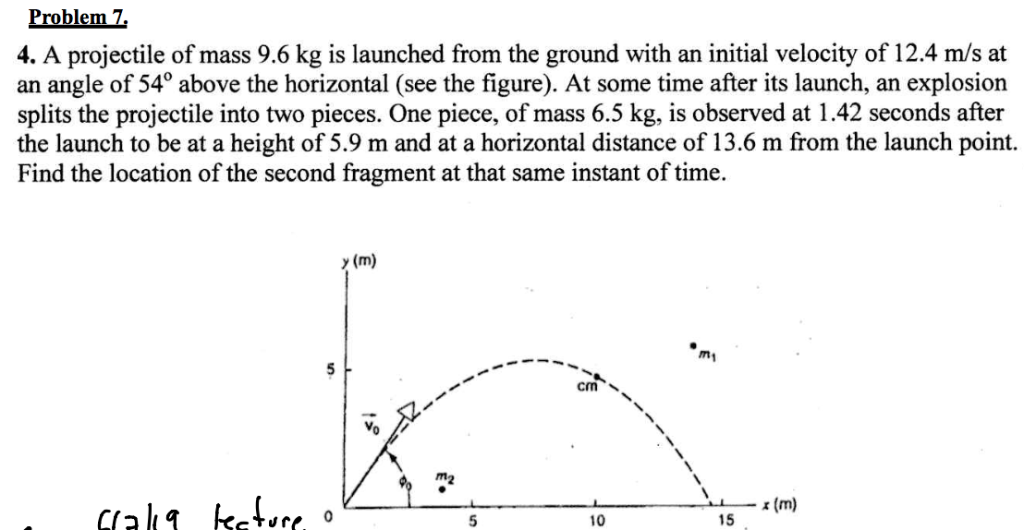Problem 7 4. A projectile of mass 9.6 kg is launched from the ground with an initial velocity of 12.4 m/s at angle of 54° above the horizontal (see the figure). At some time after its launch, an explosion splits the projectile into two pieces. One piece, of mass 6.5 kg, is observed at 1.42 seconds after the launch to be at a height of 5.9 m and at a horizontal distance of 13.6 m from the launch point Find...

• ### A projectile is fired with an initial speed of 250 m/s and angle of elevation 60°

A projectile is fired with an initial speed of 250 m/s and angle of elevation 60°. (Recall g ≈ 9.8 m/s2. Round your answers to the nearest whole number.) (a) Find the range of the projectile. (b) Find the maximum height reached. (c) Find the speed at impact.

• ### A projectile is fired with an initial speed of 250 m/s and angle of elevation 60°.

A projectile is fired with an initial speed of 250 m/s and angle of elevation 60°. The projectile is fired from a position 100 m above the ground. (Recall g ≈ 9.8 m/s2. Round your answers to the nearest whole number.) (a) Find the range of the projectile. (b) Find the maximum height reached. (c) Find the speed at impact.

• ### A projectile is launched from level ground at an angle of 30 degrees to the horizontal....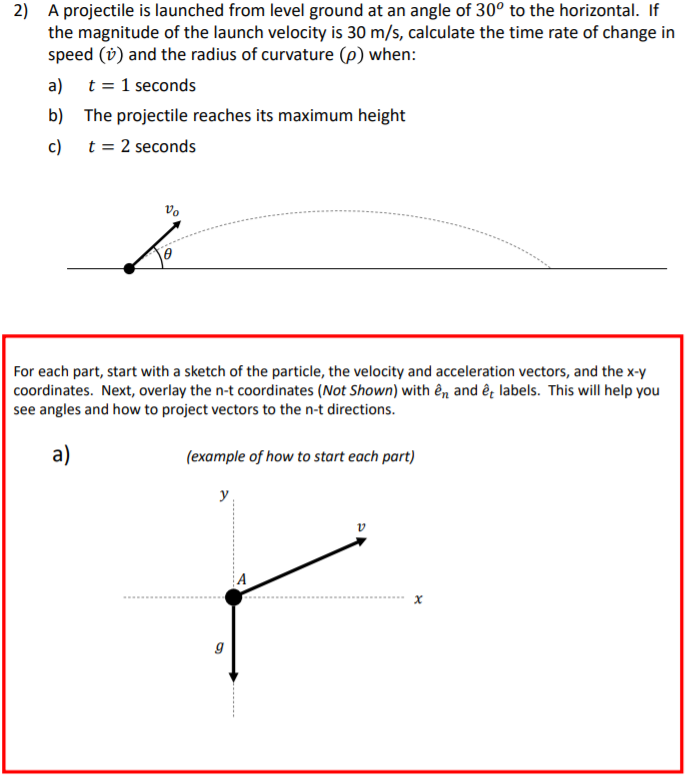A projectile is launched from level ground at an angle of 30 degrees to the horizontal. If the magnitude of the launch velocity is 30 m/s, calculate the time rate of change in speed and radius of curvature when t=1, 2, and when the projectile is at its max height. Please do the problem how the description says to do it below and put the answer neatly in a table format. Thank you. 2) A projectile is launched from level...

• ### 2. A rocket of mass m is fired vertically from the Earth, with an initial speed U. It rises to a ...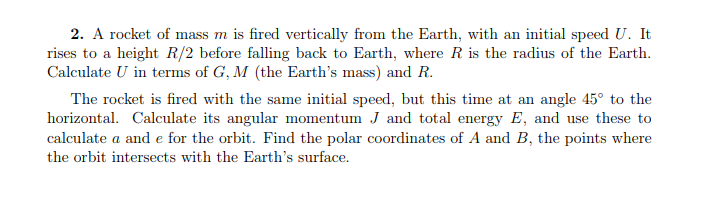2. A rocket of mass m is fired vertically from the Earth, with an initial speed U. It rises to a height R/2 before falling back to Earth, where R is the radius of the Earth. Calculate U in terms of G, M (the Earth's mass) and R. The rocket is fired with the same initial speed, but this time at an angle 45° to the horizontal. Calculate its angular momentum J and total energy E, and use these to...

• ### A projectile is launched from the top of a cliff at an angle of 45 degrees...

A projectile is launched from the top of a cliff at an angle of 45 degrees and with a velocity of 600 m/s. See Figure 1. What will be the height of the projectile at a distance of 1500 m from the launch point? What will be the distance, d1, from the launch point when the projectile will have a velocity vector at an angle of 60 degrees below the horizontal?